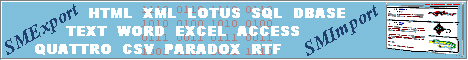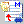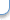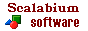Scalabium SoftwareKnowledge for your independence'.HomeDelphi and C++Builder tips

# #129: How can I calculate a checksum by modulus 10?

Today I want to publish a code for checksum calculation by modulus 10.

This algirithm is very popular for UPC barcodes (Universal Product Code), hash code or serial number generation for applications etc...

The basic algorithm:

1. add the values of the digits in the odd positions (1, 3, 5...)
2. multiply this result by 3
3. add the values of the digits in the even positions (2, 4, 6...)
4. sum the results of steps 2 and 3
5. the check digit is the smallest number which, when added to the result in step 4, produces a multiple of 10.

Small example. Assume the source data is 08137919805

1. 0+1+7+8+5=22
2. 22*3=66
3. 8+3+9+9+0=29
4. 66+29=95
5. 95+??=100 where ?? is a 5 (our checksum)

My implementation in the Pascal:

```function Mod10(const Value: string): Integer;
var
i, intOdd, intEven: Integer;
begin
{add all odd seq numbers}
intOdd := 0;
i := 1;
while (i < Length(Value)) do
begin
Inc(intOdd, StrToIntDef(Value[i], 0));
Inc(i, 2);
end;

{add all even seq numbers}
intEven := 0;
i := 2;
while (i < Length(Value)) do
begin
Inc(intEven, StrToIntDef(Value[i], 0));
Inc(i, 2);
end;

Result := 3*intOdd + intEven;
{modulus by 10 to get}
Result := Result mod 10;
if Result <> 0 then
Result := 10 - Result
end;
```

You can expand this algorithm for own needs.

For example, I modified it and\par now I use it for any characters (not only digits) in source value.
The original algorithm I use for UPC-barcode validation in the SMReport Designer and the my extended algorithm I use in the serial number generation as part of the protection schema (in the shareware projects).

Published: October 24, 2001See alsoExcel Reader (dll)ABA Picture ConvertViewer for MS Outlook MessagesExcelFile ViewerWord Web-streamMetafile ConvertABA Spreadsheet ConvertParadox ActiveXViewer for TNEF-files (winmail.dat)Clarion to Text converterContact to webmasterCopyright© 1998-2021, Scalabium Software. All rights reserved. webmaster@scalabium.com